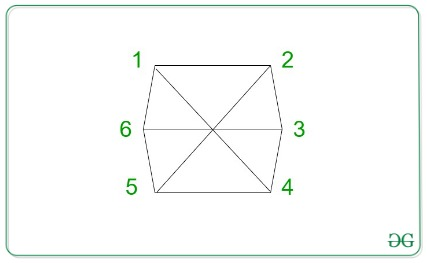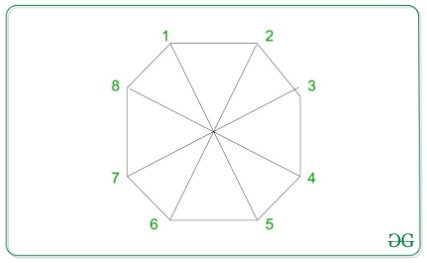# Find the vertex diagonally opposite to the vertex M from an N-sided polygon

Given two integers N and M, the task is to find the vertex diagonally opposite to the Mth vertex of an N-sided polygon.

Examples:

Input: N = 6, M = 2
Output:
Explanation:It can be observed from the image above that the vertex opposite to vertex 5 is 2.

Input: N = 8, M = 5
Output:
Explanation:It can be observed from the image above that the vertex opposite to vertex 8 is 1.

Approach: The following two cases need to be considered to solve the given problem:

1. If M > N / 2: The vertex will always be M — (N / 2).
2. If M ≤ N / 2: The vertex will always be M + (N / 2).

Below is the implementation of the above approach:

## C++

 `// C++ program for the above approach`   `#include ` `using` `namespace` `std;`   `// Function to return the` `// required vertex` `int` `getPosition(``int` `N, ``int` `M)` `{`   `    ``// Case 1:` `    ``if` `(M > (N / 2)) {` `        ``return` `(M - (N / 2));` `    ``}`   `    ``// Case 2:` `    ``return` `(M + (N / 2));` `}`   `// Driver Code` `int` `main()` `{` `    ``int` `N = 8, M = 5;` `    ``cout << getPosition(N, M);`   `    ``return` `0;` `}`

## Java

 `// Java program for ` `// the above approach` `class` `GFG{`   `// Function to return the` `// required vertex` `static` `int` `getPosition(``int` `N, ` `                       ``int` `M)` `{` `  ``// Case 1:` `  ``if` `(M > (N / ``2``)) ` `  ``{` `    ``return` `(M - (N / ``2``));` `  ``}`   `  ``// Case 2:` `  ``return` `(M + (N / ``2``));` `}`   `// Driver Code` `public` `static` `void` `main(String[] args)` `{` `  ``int` `N = ``8``, M = ``5``;` `  ``System.out.print(getPosition(N, M));`   `}` `}`   `// This code is contributed by Rajput-Ji`

## Python3

 `# Python3 program for the ` `# above approach`   `# Function to return the ` `# required vertex ` `def` `getPosition(N, M): ` `    `  `  ``# Case 1: ` `  ``if` `(M > (N ``/``/` `2``)): ` `    ``return` `(M ``-` `(N ``/``/` `2``)) ` `   `  `  ``# Case 2: ` `  ``return` `(M ``+` `(N ``/``/` `2``)) ` `  `  `# Driver Code ` `N ``=` `8` `M ``=` `5`   `print``(getPosition(N, M)) `   `# This code is contributed by code_hunt`

## C#

 `// C# program for the above approach` `using` `System;`   `class` `GFG{`   `// Function to return the` `// required vertex` `static` `int` `getPosition(``int` `N, ``int` `M)` `{` `    `  `    ``// Case 1:` `    ``if` `(M > (N / 2)) ` `    ``{` `        ``return` `(M - (N / 2));` `    ``}` `    `  `    ``// Case 2:` `    ``return` `(M + (N / 2));` `}`   `// Driver Code` `public` `static` `void` `Main(String[] args)` `{` `    ``int` `N = 8, M = 5;` `    `  `    ``Console.Write(getPosition(N, M));` `}` `}`   `// This code is contributed by Amit Katiyar`

Output:

```1

```

Time Complexity: O(1)
Auxiliary Space: O(1)

Attention reader! Don’t stop learning now. Get hold of all the important DSA concepts with the DSA Self Paced Course at a student-friendly price and become industry ready.

My Personal Notes arrow_drop_upCheck out this Author's contributed articles.

If you like GeeksforGeeks and would like to contribute, you can also write an article using contribute.geeksforgeeks.org or mail your article to contribute@geeksforgeeks.org. See your article appearing on the GeeksforGeeks main page and help other Geeks.

Please Improve this article if you find anything incorrect by clicking on the "Improve Article" button below.